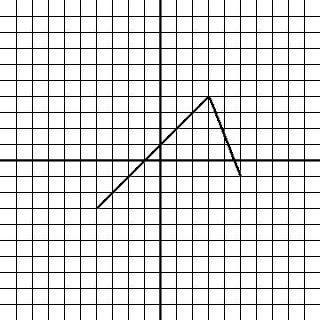# Piecewise Functions Explained

The last post introduced you to functions and how they work, but there are few other things worth discussing - first I'll talk a bit more about function notations, and then after that, I will go over piecewise functions.

When I introduced functions, I referred to the standard notation as f(x). However, you will quickly come across problems that use different letters, but they mean the same things. You will probably see things like g(x) or h(x), but they are just used to differentiate between different functions. An example will probably clear this up:

f(x) = 2x + 3
g(x) = x - 8
h(x) = 4x + 1

That shows that function f (or g, or h) is equal to the given expression. So f(x), g(x), and h(x) all represent different functions. It's the equivalent of saying 3x = 12, or 5a = 10... the math is the same, just with different letters.

So, with multiple expressions, we can do slightly more complicated things. If we say solve f(1), we substitute 1 in for x, and solve it to get 5:

f(x) = 2x + 3
f(1) = 2(1) + 3
f(1) = 5

However, we can now ask for something like f(g(x))... read as "f of 'g of x'." Looks complicated, but keeping in mind what the function notation is saying, we can easily solve this by substituting the expression for g(x) in for x (instead of just subbing in a single number like before):

f(x) = 2x + 3, g(x) = x - 8
f(g(x)) = 2(x - 8) + 3
f(g(x)) = 2x - 16 + 3
f(g(x)) = 2x - 13

Furthermore, if we wanted to solve f(g(5)), we can do one of two things. We can evaluate the expression as we just did, then solve when x= 5... or we can solve g(5) first, then substitute that into f(g(x)):

Method 1:
f(g(x)) = 2x - 13
f(g(5)) = 2(5) - 13
f(g(5)) = (-3)

Method 2:
g(x) = x - 8
g(5) = 5 - 8
g(5) = (-3)...
then...
f(x) = 2x + 3
f(g(5)) = f(-3) = 2(-3) + 3
f(g(5)) = (-3)

Both methods get the same answer, because they are doing the same things. It's just a matter of what you are more comfortable with. Function notation can get very confusing like this, but for problems like this, you just have to basically work from the inside out, just as in any other math expression you've seen! (eg. (2x + 7(3x -4) + 2).... solve the inside bracket first, then the outside). They may seem tricky, but you will be surprised at how easy they are when you see through the notation and know what they are asking!

Another point to discuss is functions that are not described by a single equation, but remain continuous. Remember back to what we said a function is... a way of relating two variables, where each number in the domain corresponds to ONLY ONE number in the range. Also remember the vertical line test. But we never said anything about it being entirely expressed by one equation! So then, we can say that the following is also a function:Obviously, it is composed of two straight lines, each having their own equation. This is called a piecewise function. Since it is just one line with two distinct 'pieces,' it is a single function, not two, and it is written as:
That says that f(x) is described by two separate equations, over the domains of x stated after each equation.  Given this graph or equation, if you were then asked to solve f(2), you must look to see within which domain x = 2 falls, and evaluate that expression. For this example, f(2) is in the top equation, so f(2) = 3. You can check by looking at the graph to see that when x = 2, the function evaluates to (y equals) 3. Alternately, f(4) is within the bottom expression, so f(4) =3/2.

Try to remember that as difficult and tricky as some functions look, they aren't that difficult if you relate to them what you know already about solving math problems and you pay attention to what is being asked! Remember, if you need something clarified further, don't hesitate to post a comment!